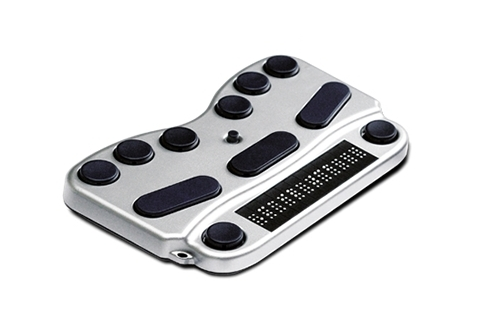Harpo BraillePen 14 Button Assignments

The default button assignments for the Harpo BraillePen 14 are listed below.You can also discover what your Braille display buttons do by using SuperNova's and ScreenReader's Key Describe Modes.

To use Key Describe Mode, simply press CAPS LOCK + FORWARD SLASH and then press the buttons or button combinations on the display to find out what they do.

To leave Key Describe Mode, press CAPS LOCK + FORWARD SLASH again.

## Action:

Open Control Panel =DOT 1+DOT 3+DOT 5+SPACE

Open Control Panel Popup Menu =CMD_HOTKEY_POPUP_MENU=DOT 1+DOT 2+DOT 3+DOT 4+SPACE

List System Tray =DOT 2+DOT 3+DOT 4+DOT 5+DOT 7+SPACE

## Text Input:

Text Input Left one character=DOT 3+SPACE

Text Input Left one character=JOYSTICK LEFT

Text Input Right one character=DOT 6+SPACE

Text Input Right one character =JOYSTICK RIGHT

Text Input Move to start of line=DOT 1+DOT 2+DOT 3+DOT 7+SPACE

Text Input Move to start of line=JOYSTICK LEFT+SPACE

Text Input Move to end of line=DOT 4+DOT 5+DOT 6+DOT 7+SPACE

Text Input Move to end of line=JOYSTICK RIGHT+SPACE

## Dolphin Cursor:

Dolphin Cursor (Movement) Right Word=DOT 5+SPACE

Dolphin Cursor (Movement) Right Word=DOT 8+JOYSTICK RIGHT

Dolphin Cursor (Movement) Left Word=DOT 2+SPACE

Dolphin Cursor (Movement) Left Word=DOT 8+JOYSTICK LEFT

Dolphin Cursor (Movement) Start of line=DOT 1+DOT 2+DOT 3+DOT 7+SPACE

Dolphin Cursor (Movement) Start of line=JOYSTICK LEFT+SPACE

Dolphin Cursor (Movement) End of line=DOT 4+DOT 5+DOT 6+DOT 7+SPACE

Dolphin Cursor (Movement) End of line=JOYSTICK RIGHT+SPACE

Dolphin Cursor (Movement) Next Line=JOYSTICK DOWN

Dolphin Cursor (Movement) Previous Line=JOYSTICK UP

Dolphin Cursor (Movement) Previous Line=DOT 1+SPACE

Dolphin Cursor (Movement) Previous Line=JOYSTICK UP

Dolphin Cursor (Movement) Right=JOYSTICK RIGHT

Dolphin Cursor (Movement) Right=DOT 6+SPACE

Dolphin Cursor (Movement) Right=JOYSTICK RIGHT

Dolphin Cursor (Movement) Left=JOYSTICK LEFT

Dolphin Cursor (Movement) Left=DOT 3+SPACE

Dolphin Cursor (Movement) Left=JOYSTICK LEFT

Dolphin Cursor (General) Turn Dolphin Cursor off=DOT 1+DOT 2+DOT 4+SPACE

Dolphin Cursor (Movement) Next Object=DOT 5+DOT 6+SPACE

Dolphin Cursor (Movement) Next Object=DOT 5+DOT 6+DOT 7+SPACE

Dolphin Cursor (Movement) Previous Object=DOT 2+DOT 3+SPACE

Dolphin Cursor (Movement) Move Dolphin Cursor to next Window=DOT 5+DOT 6+DOT 8+SPACE

Dolphin Cursor (Movement) Move Dolphin Cursor to previous Window=DOT 2+DOT 3+DOT 8+SPACE

Dolphin Cursor (Movement) Top of Window / Area=DOT 1+DOT 2+DOT 3+SPACE

Dolphin Cursor (Movement) Bottom of Window / Area=DOT 4+DOT 5+DOT 6+SPACE

Dolphin Cursor (General) Forms Mode toggle=DOT 4+DOT 6+DOT 8+SPACE

Dolphin Cursor (Movement) Next Large Object=DOT 3+DOT 4+DOT 5+SPACE

Dolphin Cursor (Movement) Next Large Object=JOYSTICK DOWN+SPACE

Dolphin Cursor (Movement) Previous Large Object=DOT 1+DOT 2+DOT 6+SPACE

Dolphin Cursor (Movement) Previous Large Object=JOYSTICK UP+SPACE

Dolphin Cursor (General) Find with Dolphin Cursor=DOT 1+DOT 2+DOT 4+DOT 8

Dolphin Cursor (General) Find Next with Dolphin Cursor=DOT 1+DOT 3+DOT 4+DOT 5+DOT 8

Dolphin Cursor (General) Find Previous with Dolphin Cursor=DOT 1+DOT 2+DOT 3+DOT 4+DOT 8

## Speak Keys:

Speak Keys Character (MultiKey)=DOT 3+DOT 6+SPACE

Speak Keys Word (MultiKey)=DOT 2+DOT 5+SPACE

Speak Keys Line (MultiKey)=DOT 1+DOT 4+SPACE

Speak Keys Status (Multikey)=DOT 2+DOT 3+DOT 7+SPACE

Speak Keys Selection (Multikey)=DOT 2+DOT 7+SPACE

Speak Keys Focus (MultiKey)=DOT 3+DOT 5+DOT 6+DOT 7+SPACE

Speak Keys Headings (MultiKey)=DOT 2+DOT 5+DOT 7+SPACE

Speak Keys Control (MultiKey)=DOT 4+DOT 6+DOT 7+SPACE

Speak Keys Window (MultiKey)=DOT 3+DOT 5+DOT 7+SPACE

Speak Keys Where am I?=DOT 2+DOT 4+SPACE

Speak Keys Describe Focus position (Multikey)=DOT 2+DOT 3+DOT 6+DOT 7+SPACE

Speak Keys Word Move to Next=DOT 5+SPACE

Speak Keys Word Move to Next=DOT 8+JOYSTICK RIGHT

Speak Keys Word Move to Previous=DOT 2+SPACE

Speak Keys Word Move to Previous=DOT 8+JOYSTICK LEFT

## Braille:

Braille Cycle Attributes=DOT 7+SPACE

Braille Describe Character on off=DOT 8+SPACE

Braille Width Left=LEFT THUMB

Braille Width Right=RIGHT THUMB

Braille Half Width Left=DOT 7+LEFT THUMB

Braille Half Width Right=DOT 7+RIGHT THUMB

Braille Line Start=DOT 8+LEFT THUMB

Braille Line End=DOT 8+RIGHT THUMB

Braille Line down=DOT 7+JOYSTICK DOWN

Braille Line up=DOT 7+JOYSTICK UP

Braille Bottom=DOT 8+JOYSTICK DOWN

Braille Top=DOT 8+JOYSTICK UP

## Simulate Key Press:

Simulate Key Press Up=JOYSTICK UP

Simulate Key Press Up=DOT 1+SPACE

Simulate Key Press Up=JOYSTICK UP

Simulate Key Press Down=JOYSTICK DOWN

Simulate Key Press Down=DOT 4+SPACE

Simulate Key Press Down=JOYSTICK DOWN

Simulate Key Press Left=JOYSTICK LEFT

Simulate Key Press Left=DOT 3+SPACE

Simulate Key Press Left=JOYSTICK LEFT

Simulate Key Press Right=JOYSTICK RIGHT

Simulate Key Press Right=DOT 6+SPACE

Simulate Key Press Right=JOYSTICK RIGHT

Simulate Key Press Home=DOT 1+DOT 2+DOT 3+DOT 7+SPACE

Simulate Key Press Home=JOYSTICK LEFT+SPACE

Simulate Key Press End=DOT 4+DOT 5+DOT 6+DOT 7+SPACE

Simulate Key Press End=JOYSTICK RIGHT+SPACE

Simulate Key Press Enter=JOYSTICK ACTION

Simulate Key Press Enter=DOT 8

Simulate Key Press Enter=DOT 4+DOT 6+SPACE

Simulate Key Press Enter=JOYSTICK ACTION

Simulate Key Press Space=SPACE

Simulate Key Press Page Up=DOT 1+DOT 2+DOT 6+SPACE

Simulate Key Press Page Up=JOYSTICK UP+SPACE

Simulate Key Press Page Down=DOT 3+DOT 4+DOT 5+SPACE

Simulate Key Press Page Down=JOYSTICK DOWN+SPACE

Simulate Key Press Tab=DOT 5+DOT 6+SPACE

Simulate Key Press Tab=DOT 5+DOT 6+DOT 7+SPACE

Simulate Key Press Shift Tab=DOT 2+DOT 3+SPACE

Simulate Key Press Ctrl Tab=DOT 5+DOT 6+DOT 8+SPACE

Simulate Key Press Ctrl Shift Tab=DOT 2+DOT 3+DOT 7+DOT 8+SPACE

Simulate Key Press Escape=DOT 1+DOT 5+SPACE

Simulate Key Press Backspace=DOT 7

Simulate Key Press Backspace=DOT 1+DOT 2+SPACE

Simulate Key Press Delete=DOT 1+DOT 4+DOT 5+SPACE

Simulate Key Press Windows=DOT 2+DOT 4+DOT 5+DOT 6+SPACE

Simulate Key Press Ctrl Home=DOT 1+DOT 2+DOT 3+SPACE

Simulate Key Press Ctrl End=DOT 4+DOT 5+DOT 6+SPACE